# An Introduction to Evaluating Structural Model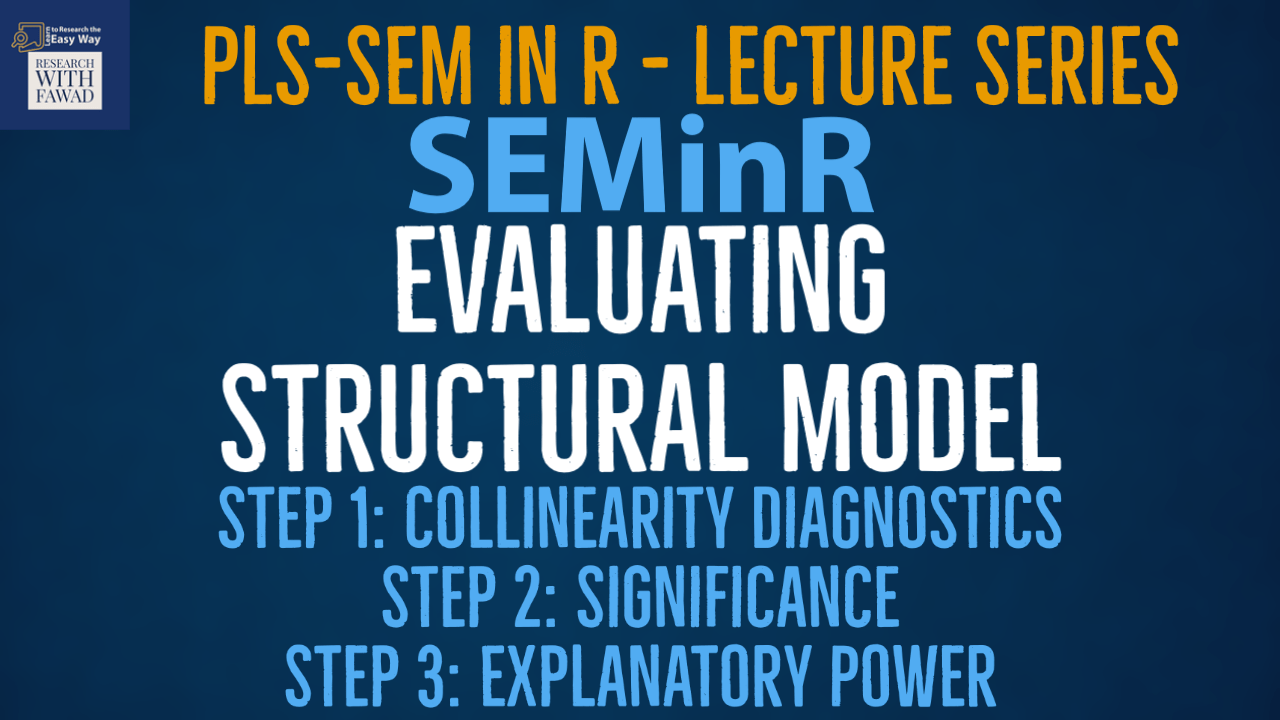### SEMinR Lecture Series

This session is focused on evaluating structural model. The session focuses on the three steps.
Step 1: Collinearity Diagnostics
Step 2: Structural Relationships
Step 3: Explanatory Power (R-Square and F-Square)

## Evaluating Structural Model

• Once you have confirmed that the measurement of constructs is reliable and valid, the next step addresses the assessment of the structural model results.
• Figure shows a systematic approach to the structural model assessment.
• In the first step, you need to examine the structural model for potential collinearity issues.
• The reason is that the estimation of path coefficients in the structural models is based on ordinary least squares (OLS) regressions of each endogenous construct on its corresponding predictor constructs. The path coefficients might be biased if the estimation involves high levels of collinearity among predictor constructs.
• Once you have ensured that collinearity is not a problem, you will evaluate the significance and relevance of the structural model relationships (i.e., the path coefficients).
• Steps 3 and 4 of the procedure involve examining the model’s explanatory power and predictive power.
• In addition, some research situations involve the computation and comparison of alternative models, which can emerge from different theories or contexts. As model comparisons are not relevant for every PLS-SEM analysis, Step 5 will be performed later in the series.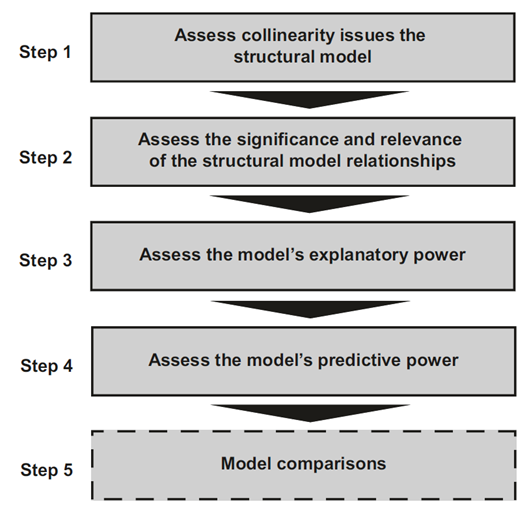## Step 1

• Structural model coefficients for the relationships between constructs are derived from estimating a series of regression equations. As the point estimates and standard errors can be biased by strong correlations of each set of predictor constructs (Sarstedt & Mooi, 2019; Chap. 7), the structural model regressions must be examined for potential collinearity issues.
• This process is similar to assessing formative measurement models, but in this case, the construct scores of the predictor constructs in each regression in the structural model are used to calculate the variance inflation factor (VIF) values.
• VIF values above 5 are indicative of probable collinearity issues among predictor constructs, but collinearity can also occur at lower VIF values of 3–5 (Becker, Ringle, Sarstedt, & Völckner, 2015; Mason & Perreault, 1991).
• If collinearity is a problem, a frequently used option is to create higher-order constructs (Hair, Risher, Sarstedt, & Ringle, 2019; Hair, Sarstedt, Ringle, & Gudergan, 2018; Chap. 2; Sarstedt et al., 2019).
• We follow the structural model assessment procedure (Step 1) and begin with an evaluation of the collinearity of predictor constructs in relation to each endogenous construct.
• I examine the VIF values for the predictor constructs by inspecting the vif_antecedents element within the summary_simple object:

## The Code

``````# Inspect the structural model collinearity VIF
summary_simple\$vif_antecedents``````

## Results

• As can be seen in Fig, all VIF values are clearly below the threshold of 5. It can be concluded that collinearity among predictor constructs is likely not a critical issue in the structural model, and we can continue examining the result report.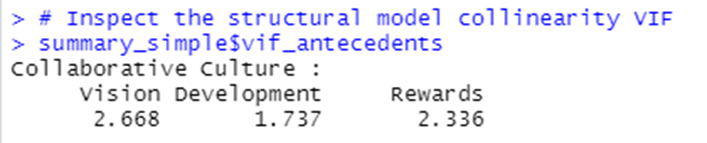## Step 2: Assess the Significance and Relevance of the Structural Model Relationships

• In the next step, the significance of the path coefficients and relevance of the path coefficients are evaluated.
• A path coefficient is significant at the 5% level if the value zero does not fall into the 95% confidence interval.
• In terms of relevance, path coefficients are usually between −1 and +1, with coefficients closer to −1 representing strong negative relationships and those closer to +1 indicating strong positive relationships.
• Note that values below −1 and above +1 may technically occur, for instance, when collinearity is at very high levels.
• Path coefficients larger than +/−1 are not acceptable, and multicollinearity reduction methods must be implemented.
• As PLS-SEM processes standardized data, the path coefficients indicate the changes in an endogenous construct’s values that are associated with standard deviation unit changes in a certain predictor construct, holding all other predictor constructs constant.
• For example, a path coefficient of 0.505 indicates that when the predictor construct increases by one standard deviation unit, the endogenous construct will increase by 0.505 standard deviation units.
• Next, in the structural model assessment procedure (Step 2), we need to evaluate the relevance and significance of the structural paths. The results of the bootstrapping of structural paths can be accessed by inspecting the bootstrapped_paths element nested in the summary_boot object (Fig.).

## Step 2: Code

``````# Inspect the structural paths
summary_boot\$bootstrapped_paths``````

## Step 2: Results

• First, let’s consider the original path coefficient estimates (column: Original Est, Fig) for the exogenous driver constructs. For example, we find that Vision, Development and Rewards have a significant positive impact on Collaborative Culture.
• Let’s now review the results for statistical significance. Assuming a 5% significance level (as specified with the parameter alpha = 0.05 in the bootstrap_model() function), the t-values (T Stat. column, Fig.) estimated from the bootstrapping should exceed the value of 1.960 (for two tailed) and 1.645 (1 tailed).
• Also, path coefficient is significant at the 5% level since, the value zero does not fall into the 95% confidence interval. There is no zero between 2.5% CI and 97.5% CI.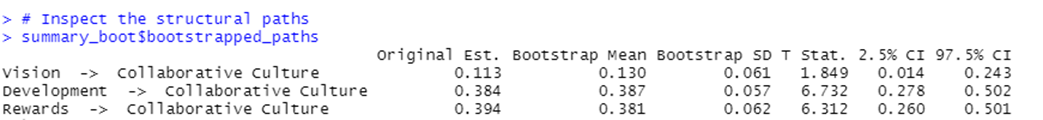## Step 3: Explanatory Power

• The next step involves examining the coefficient of determination (R-Sq) of the endogenous construct(s).
• The R-Sq represents the variance explained in each of the endogenous constructs and is a measure of the model’s explanatory power (Shmueli & Koppius, 2011), also referred to as in-sample predictive power (Rigdon, 2012). The R-Sq ranges from 0 to 1, with higher values indicating a greater explanatory power.
• As a general guideline, R-Sq values of 0.75, 0.50, and 0.25 can be considered substantial, moderate, and weak, respectively, in many social science disciplines (Hair, Ringle, & Sarstedt, 2011).
• But acceptable R-Sq values are based on the research context, and in some disciplines, an R-Sq value as low as 0.10 is considered satisfactory.
• Researchers should also be aware that R2 is a function of the number of predictor constructs – the greater the number of predictor constructs, the higher the R2.
• Therefore, the R-Sq should always be interpreted relative to the context of the study, based on the R-Sq values from related studies as well as models of similar complexity.
• A limitation of R-Sq is that the metric will tend to increase as more explanatory variables are introduced to a model.
• The adjusted R-Sq metric accounts for this by adjusting the R-Sq value based upon the number of explanatory variables in relation to the data size and is seen as a more conservative estimate of R-Sq (Theil, 1961).
• Because of the correction factor introduced to account for data and model size, the adjusted R-Sq is not a precise indication of an endogenous construct’s explained variance (Sarstedt & Mooi, 2019; Chap. 7).
• Researchers can also assess how the removal of a selected predictor construct affects an endogenous construct’s R-Sq value. This metric is the f-Sq effect size and is like the size of the path coefficients.
• In Step 3 of the structural model assessment procedure, we need to consider the model’s explanatory power by analyzing the R-Sq of the endogenous constructs and the f-Sq effect size of the predictor constructs.

## Step 3: Code

``````# Inspect the model RSquares
summary_simple\$paths
# Inspect the effect sizes
summary_simple\$fSquare``````

## Step 3: Results

• To start with, we need to examine the R-Sq values of the endogenous constructs. The R2 value of Collaborative Culture (0.608) can be considered moderate. Figure (on the right) shows the f-Sq values for all combinations of endogenous constructs (represented by the columns) and corresponding exogenous (i.e., predictor) constructs (represented by the rows).
• For example, Vision has a small effect size (0.012) whereas Development (0.214) and Rewards (0.169) have a medium effect.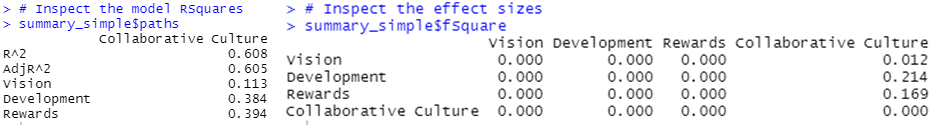## Step 1, 2, & 3: Complete Code

``````library(seminr)
# Create measurement model
simple_mm <- constructs(
composite("Vision", multi_items("VIS", 1:4), weights = mode_B),
composite("Development", multi_items("DEV", 1:7), weights = mode_B),
composite("Rewards", multi_items("RW",1:4), weights = mode_B),
composite("Collaborative Culture", multi_items("CC", 1:6)))
# Create structural model
simple_sm <- relationships(
paths(from = c("Vision","Development","Rewards"), to = "Collaborative Culture"))
# Estimate the model
simple_model <- estimate_pls(data = datas, measurement_model = simple_mm, structural_model = simple_sm, missing = mean_replacement, missing_value = "-99")
# Summarize the model results
summary_simple <- summary(simple_model)
# Iterations to converge
summary_simple\$iterations
# Bootstrap the model on the PLS Estimated Model
boot_model <- bootstrap_model(
seminr_model = simple_model,
nboot = 1000,
cores = parallel::detectCores(),
seed = 123)
# Store the summary of the bootstrapped model
# alpha sets the specified level for significance, i.e. 0.05
summary_boot <- summary(boot_model, alpha = 0.05)
# Inspect the structural model collinearity VIF
summary_simple\$vif_antecedents
# Inspect the structural paths
summary_boot\$bootstrapped_paths
# Inspect the total effects
summary_boot\$bootstrapped_total_paths
# Inspect the model RSquares
summary_simple\$paths
# Inspect the effect sizes
summary_simple\$fSquare
``````

## Reference

Hair Jr, J. F., Hult, G. T. M., Ringle, C. M., Sarstedt, M., Danks, N. P., & Ray, S. (2021). Partial Least Squares Structural Equation Modeling (PLS-SEM) Using R: A Workbook.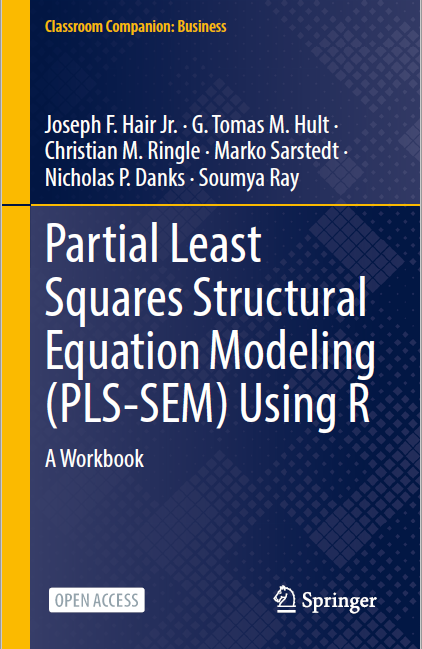The tutorials on SEMinR are based on the mentioned book. The book is open source and available for download under this link.